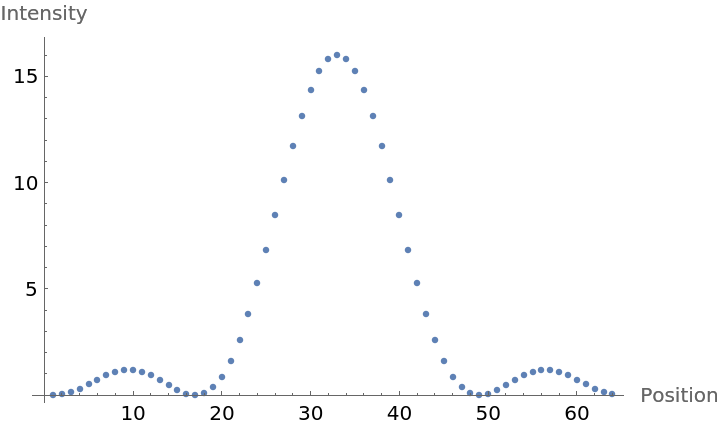Function Repository Resource:

# FastFourierGraph

Create a fast Fourier transform calculation in graphical form

 ResourceFunction["FastFourierGraph"][dim] returns an edge-weighted, time-ordered multiway graph on 2dim×2dim vertices, which describes the fast Fourier transform of a length 2dim vector. ResourceFunction["FastFourierGraph"][data] takes a list of data with length 2dim and adds it to the graph under the annotation key VertexWeight.

## Details

Edge connections and edge weights (set to the phases) are determined by recursive decomposition of FourierMatrix[2dim].
Assuming data list input, ResourceFunction["FastFourierGraph"] takes the option "Evaluate" whose default value is False.
Setting "ComputeValues"True populates values through the Graph and returns a complete evaluation through the annotation key "VertexWeight".
This technique is slow compared to Compile-based methods, but still useful for teaching purposes and complexity analysis.

## Examples

### Basic Examples (5)

Calculate the graph for the base case of the fast Fourier transform:

 In:=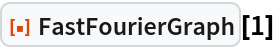Out=Plot the next induced case:

 In:=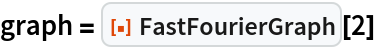Out=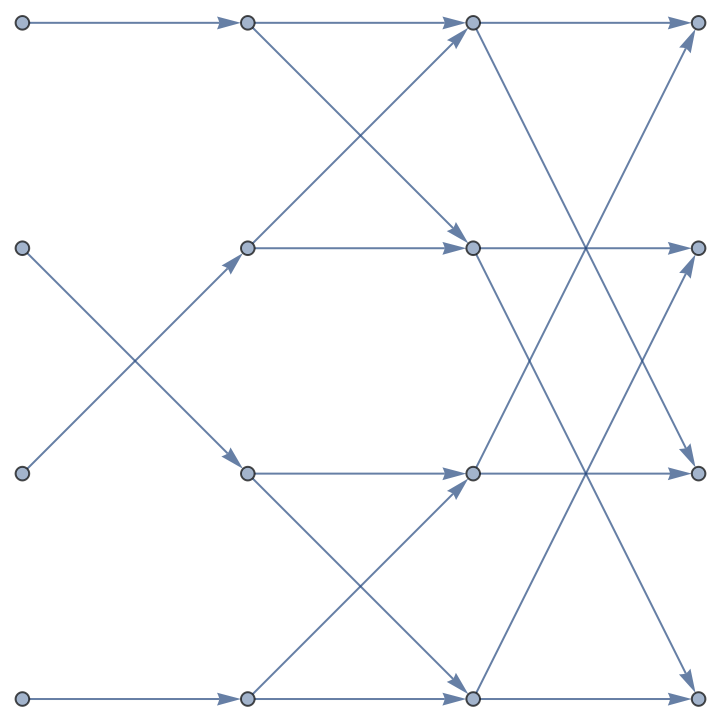Color edges according to phases:

 In:=Out=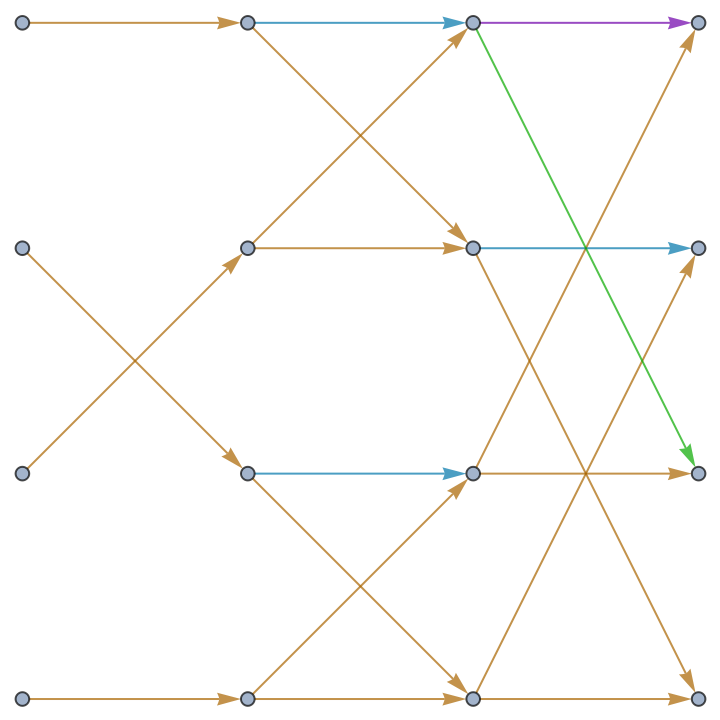Notice n log n complexity in rectangular dimensions of subsequent graphs:

 In:=Out=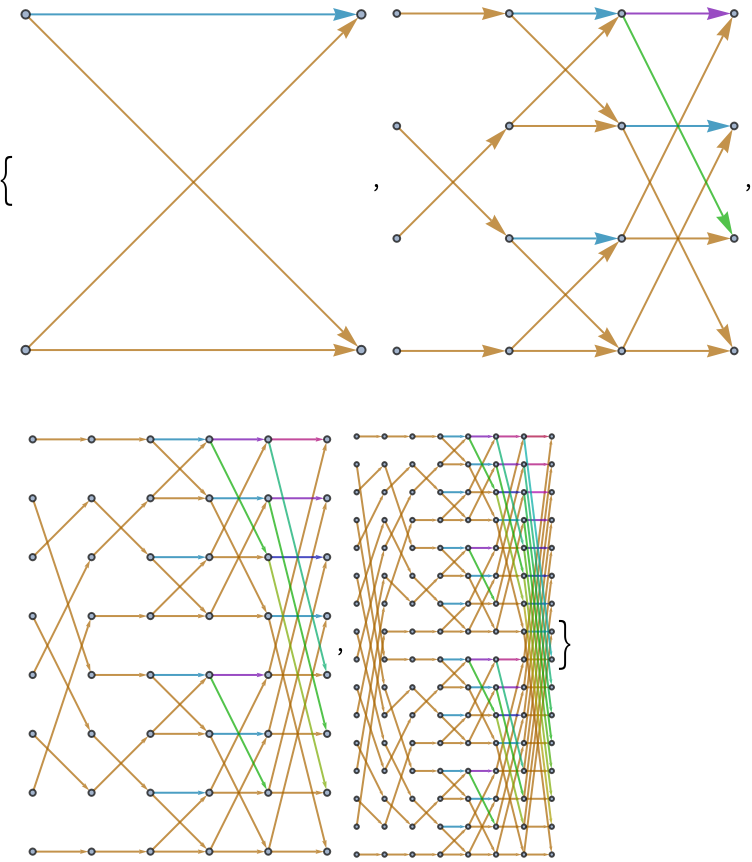Blow up the graphs to see their free-form structure using "SpringElectricalEmbedding":

 In:=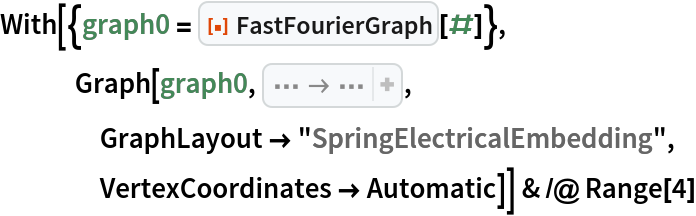Out=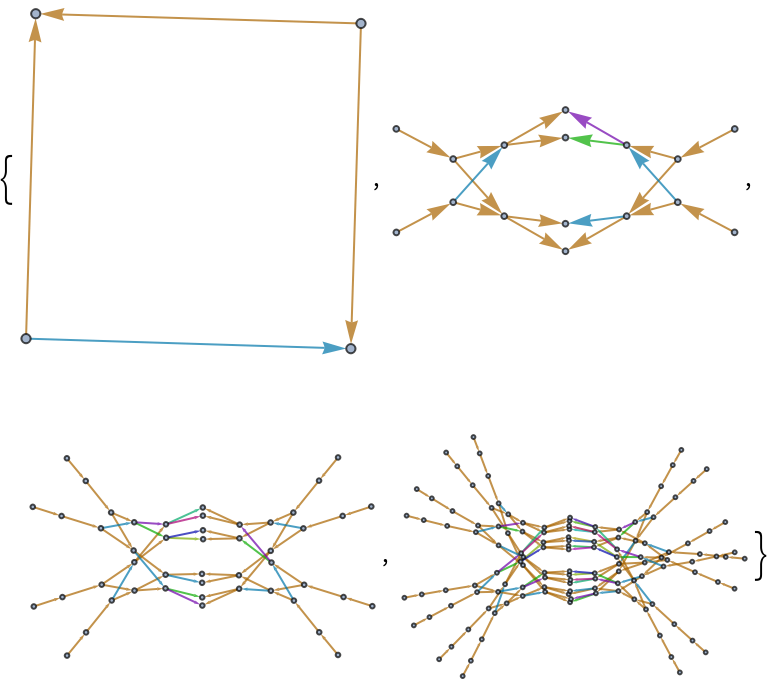Create a graph from data:

 In:=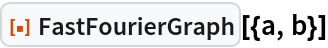Out=### Options (5)

Use the option "ComputeValues"True to compute the transformation values while generating the graph:

 In:=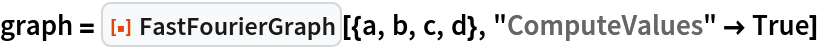Out=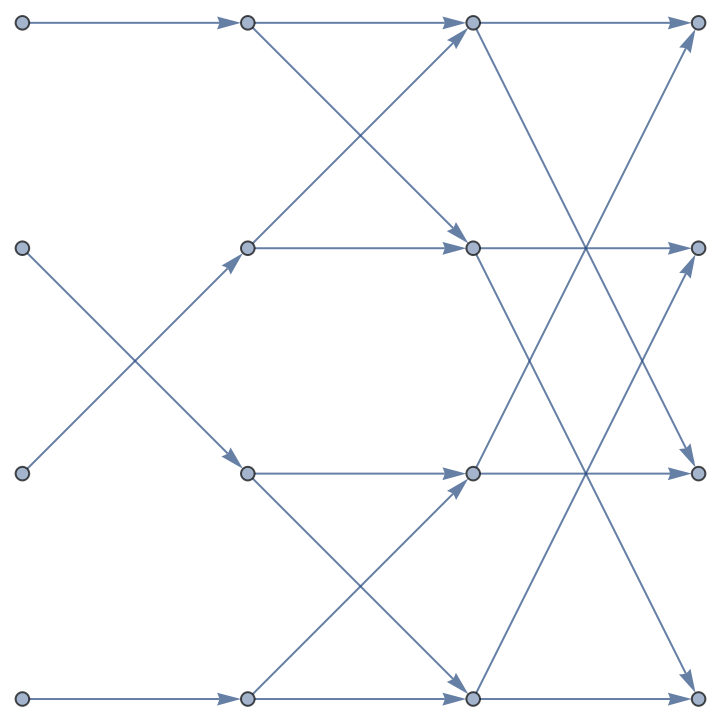See the fast Fourier transformation values by retrieving the VertexWeight:

 In:=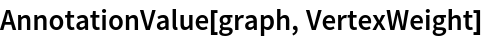Out=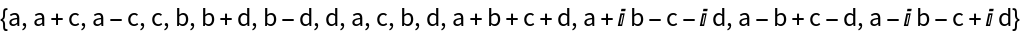Depict results of the calculation as above:

 In:=Out=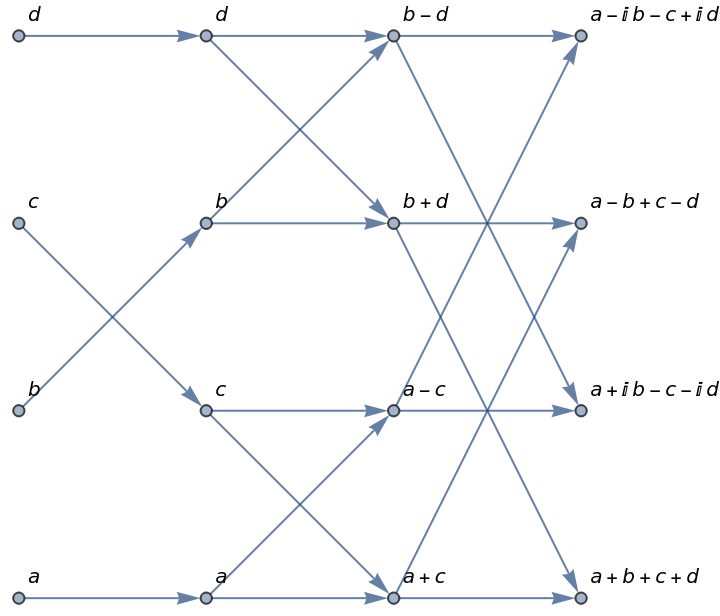Compare with the standard method using FourierMatrix:

 In:=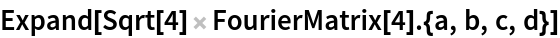Out=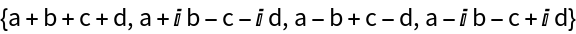Apply a similar validation technique for different input lengths:

 In:=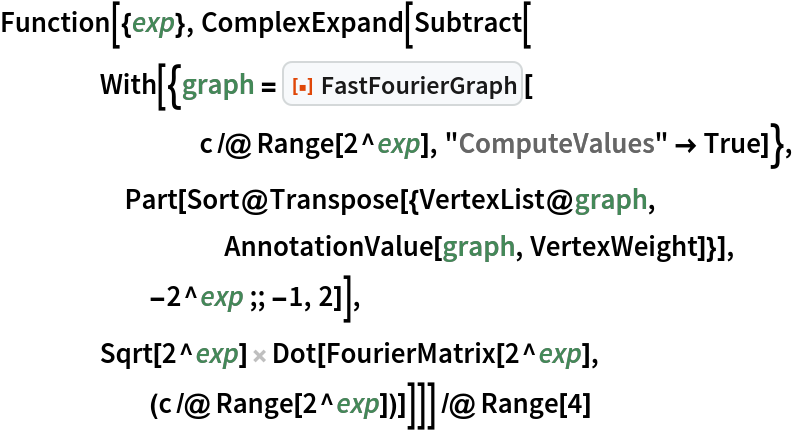Out=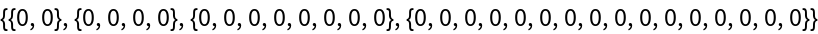### Properties and Relations (1)

Compare with ButterflyGraph:

 In:=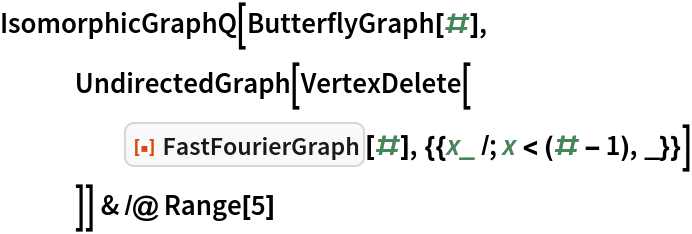Out=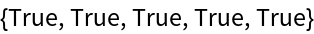### Possible Issues (2)

Input should be a list of length 2n for some integer n>0:

 In:=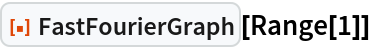Out=In:=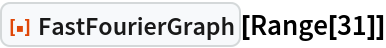Out=Timing statistics look very slow compared to the built-in Fourier:

 In:=Out=In:=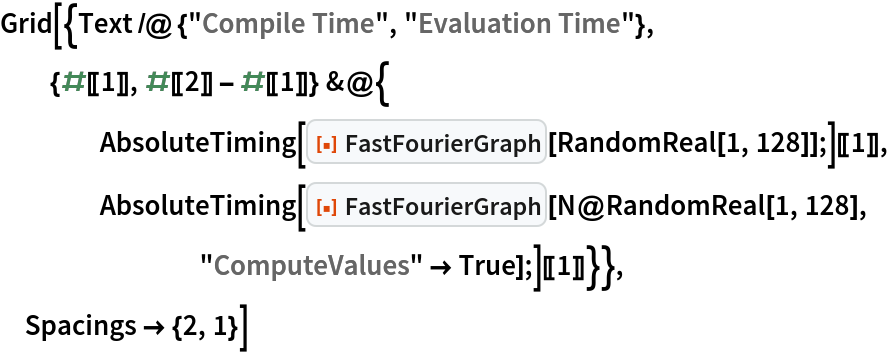Out=Compile time is relatively slow compared to ButterflyGraph:

 In:=Out=### Neat Examples (2)

Follow the different paths a value can take from input to output:

 In:=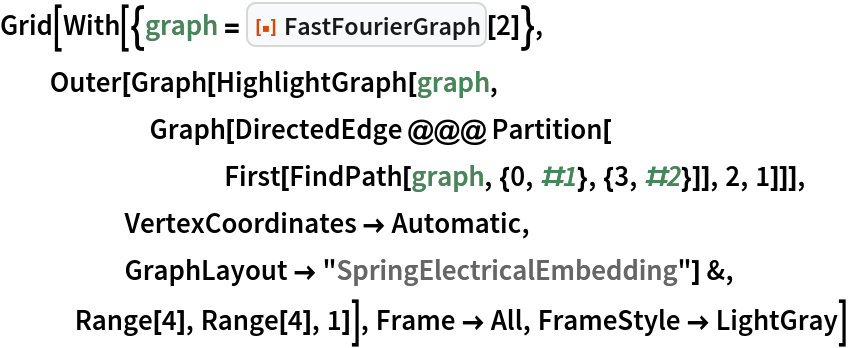Out=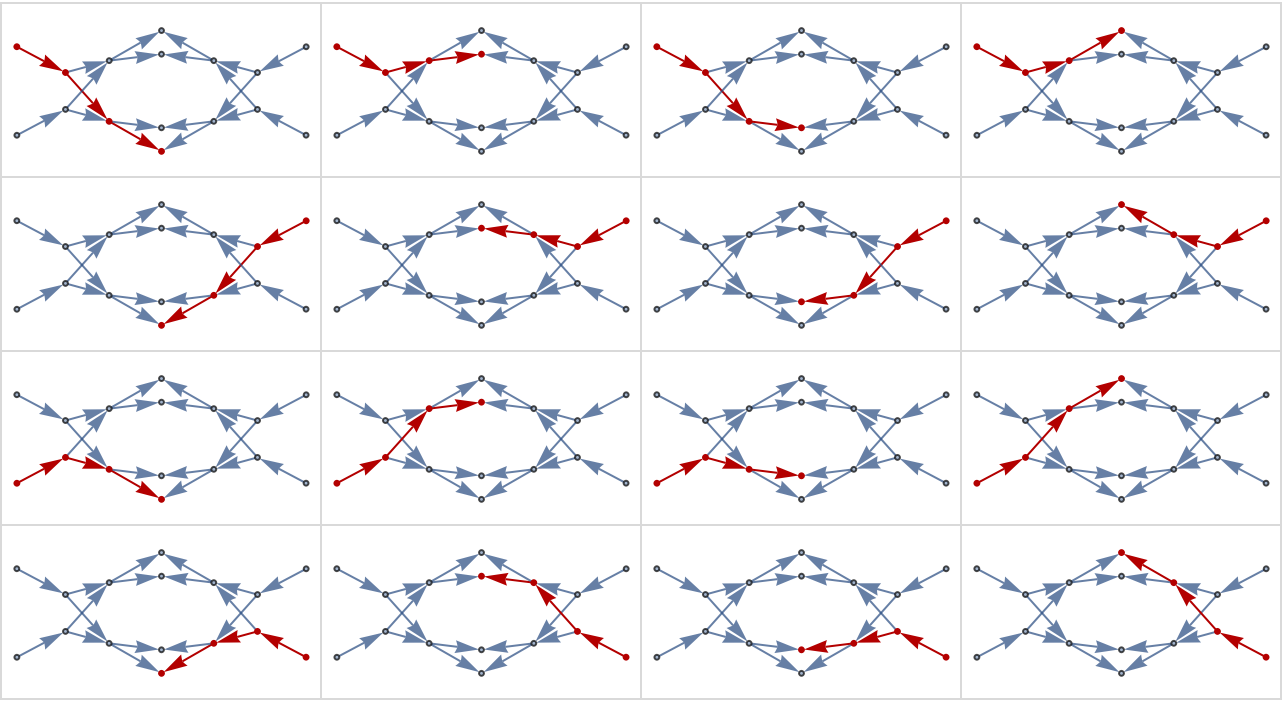Plot an intensity profile:

 In:=Out=